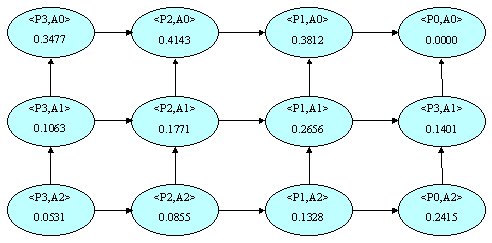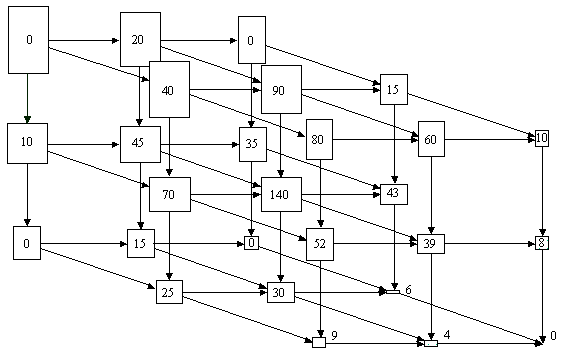# Introduction

Given a relation and a set of concept hierarchies, one for each attribute of the relation, the set of possible ways of viewing the relation during summarization (rolling-up) is called the generalization space. Assume that summarization is always done one level at a time. The Province attribute has 4 levels in its concept hierarchy, numbered P3 (for the most specific data - the original relation) to P0 (for the single value ANY). The Amount attribute also has 4 levels numbered A3 to A0. The resulting generalization space is shown in Figure 13. In Figure 13, the node labelled <P3,A3> corresponds to the original relation, as shown in Figure 3 (left hand side). The node labelled <P2,A3> corresponds to the data in Figure 5 (left hand side), and the node labelled <P2,A2> corresponds to the data in Figure 8 (left hand side). This is a 2-dimensional generalization space.

Figure 14 shows a sample 3-dimensional generalization space.Figure 13: 2-Dimensional Generalization SpaceFigure 14: 3-Dimensional Generalization Space